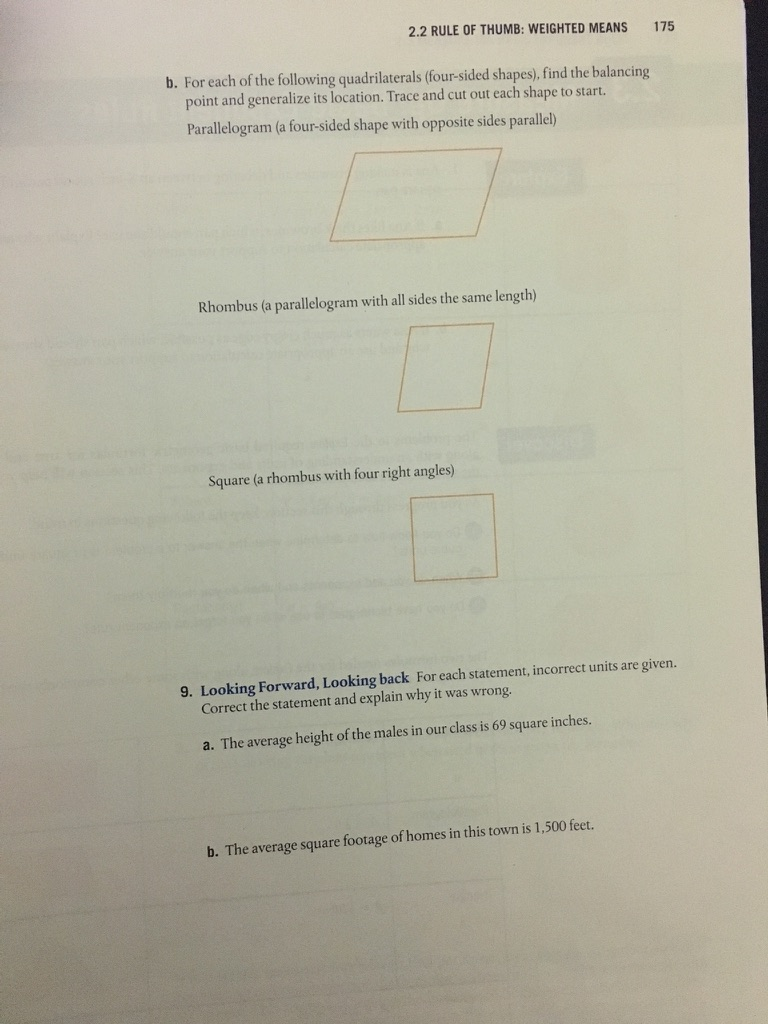# 2.2 RULE OF THUMB: WEIGHTED MEANS 175 b. For each of the following quadrilaterals (four-sided shapes), find the balancing point and generalize its location. Trace and cut out each shape to start. Parallelogram (a four-sided shape with opposite sides parallel) Rhombus (a parallelogram with all sides the same length) Square (a rhombus with four right angles) 9. Looking Forward, Looking back For each statement, incorrect units are given. Correct the statement and explain why it was wrong. a. The average height of the males in our class is 69 square inches. b. The average square footage of homes in this town is 1,500 feet.

Questionhelp_outlineImage Transcriptionclose2.2 RULE OF THUMB: WEIGHTED MEANS 175 b. For each of the following quadrilaterals (four-sided shapes), find the balancing point and generalize its location. Trace and cut out each shape to start. Parallelogram (a four-sided shape with opposite sides parallel) Rhombus (a parallelogram with all sides the same length) Square (a rhombus with four right angles) 9. Looking Forward, Looking back For each statement, incorrect units are given. Correct the statement and explain why it was wrong. a. The average height of the males in our class is 69 square inches. b. The average square footage of homes in this town is 1,500 feet. fullscreen